# Swift学习之路-高级运算符

## 位运算符

Swift 支持 C 里面所有的位运算符，具体如下：

### 位取反运算符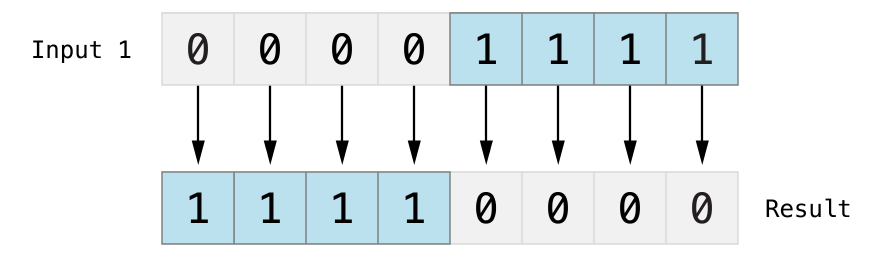``````let initialBits: UInt8 = 0b00001111
let invertedBits = ~initialBits  // equals 11110000``````

UInt8 类型的整数有八位，可以存储 0 到 255 之间的任意值。这个例子使用二进制值 00001111 初始化了一个 UInt8 类型的整数，前四位全是 0 ，后四位都是 1 。这和十进制的 15 是相等的。

### 位与运算符``````let firstSixBits: UInt8 = 0b11111100
let lastSixBits: UInt8 = 0b00111111
let middleFourBits = firstSixBits & lastSixBits // equals 00111100``````

### 位或运算符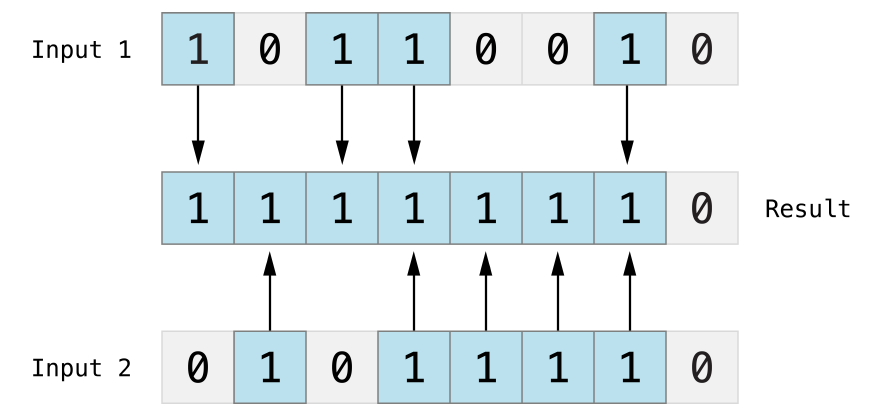``````let someBits: UInt8 = 0b10110010
let moreBits: UInt8 = 0b01011110
let combinedbits = someBits | moreBits // equals 11111110``````

### 位异或运算符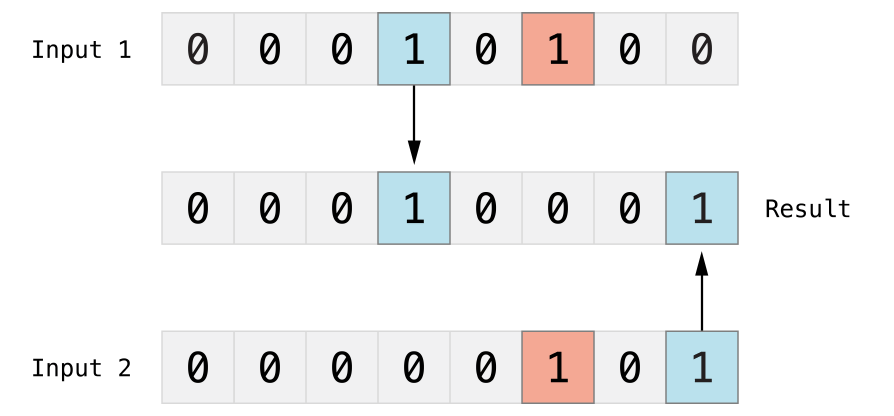``````let firstBits: UInt8 = 0b00010100
let otherBits: UInt8 = 0b00000101
let outputBits = firstBits ^ otherBits // equals 00010001``````

## 位左移和右移运算符

#### 无符号整数的移位操作

1. 已经存在的比特位按指定的位数进行左移和右移。
2. 任何移动超出整型存储边界的位都会被丢弃。
3. 用 0 来填充向左或向右移动后产生的空白位。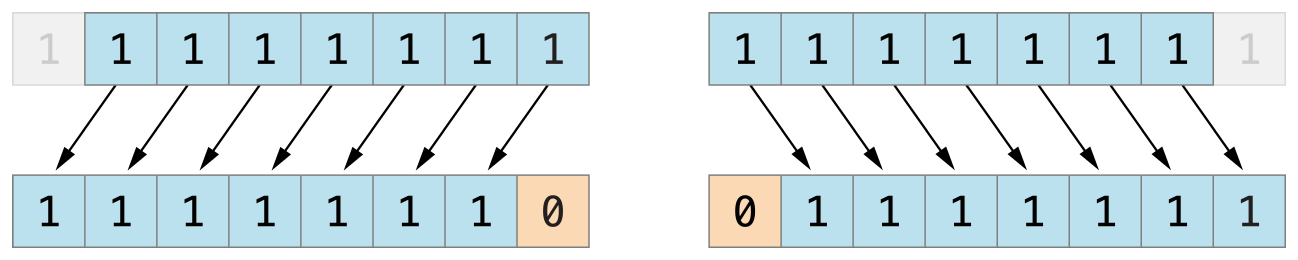``````let shiftBits: UInt8 = 4 // 00000100 in binary
shiftBits << 1 // 00001000
shiftBits << 2 // 00010000
shiftBits << 5 // 10000000
shiftBits << 6 // 00000000
shiftBits >> 2 // 00000001``````

``let pink: UInt32 = 0xCC6699 let redComponent = (pink & 0xFF0000) >> 16 // redComponent is 0xCC, or 204 let greenComponent = (pink & 0x00FF00) >> 8 // greenComponent is 0x66, or 102 let blueComponent = pink & 0x0000FF // blueComponent is 0x99, or 153``

 层叠样式表（Cascading Style Sheets），即 CSS 。

### 有符号整型的位移操作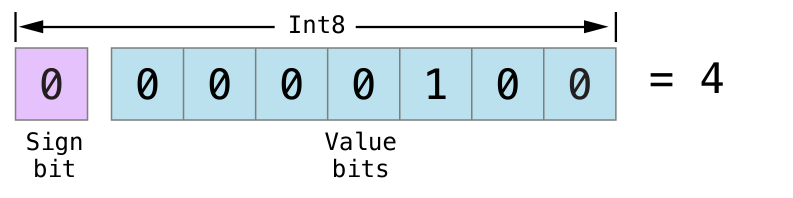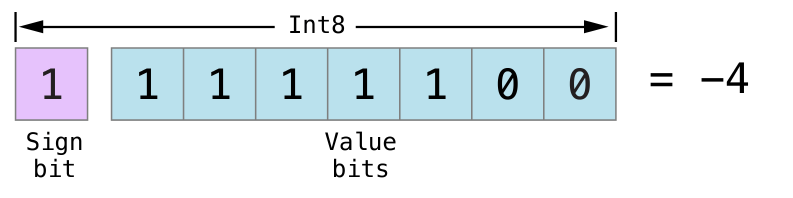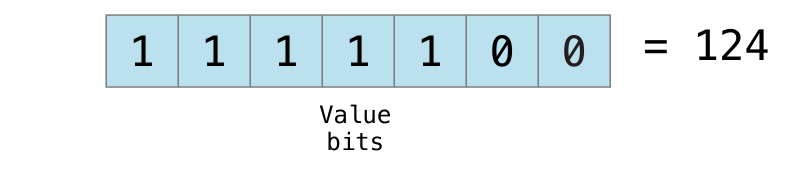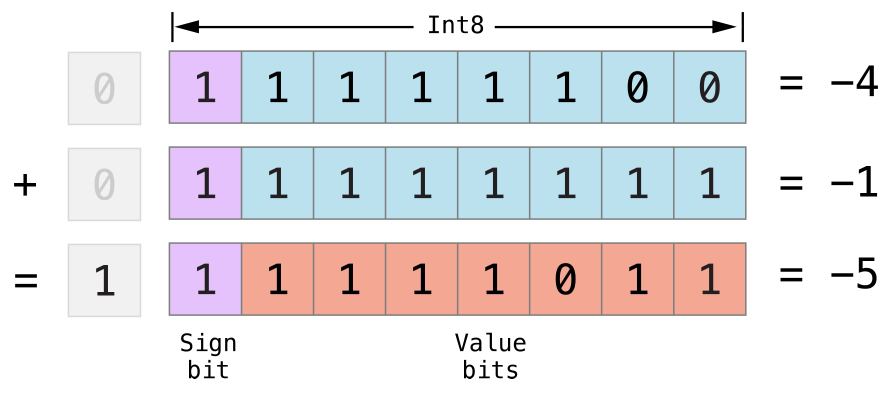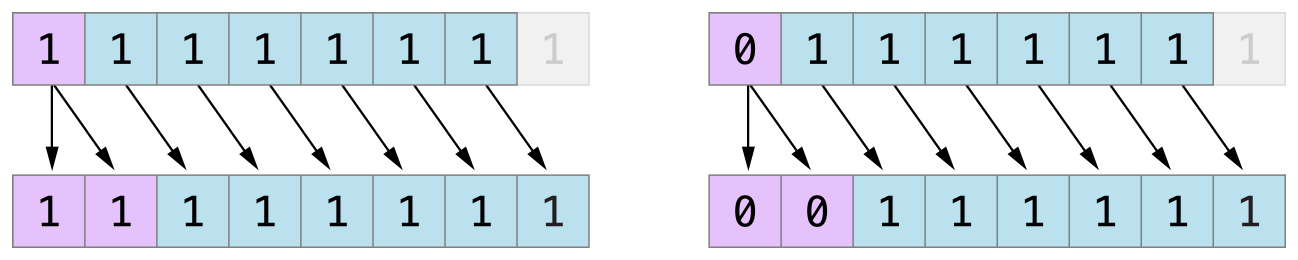## 溢出运算符

``````var potentialOverflow = Int16.max
// potentialOverflow equals 32767, which is the maximum value an Int16 can hold
potentialOverflow += 1
// this causes an error``````

• 溢出加法 （ &+ ）
• 溢出减法 （ &- ）
• 溢出乘法 （ & ）

### 值溢出

``````var unsignedOverflow = UInt8.max
// unsignedOverflow equals 255, which is the maximum value a UInt8 can hold
unsignedOverflow = unsignedOverflow &+ 1
// unsignedOverflow is now equal to 0``````

unsignedOverflow 初始化为 UInt8 所能容纳的最大整数（ 255 ，二进制为 11111111 ）。溢出加法运算符（ &+ ）对其进行加 1 操作。这使得它的二进制表示正好超出 UInt8 所能容纳的位数，也就导致它溢出了边界，如下图所示。溢出后，留在 UInt8 边界内的值是 00000000 ，也就是十进制数值的 0 。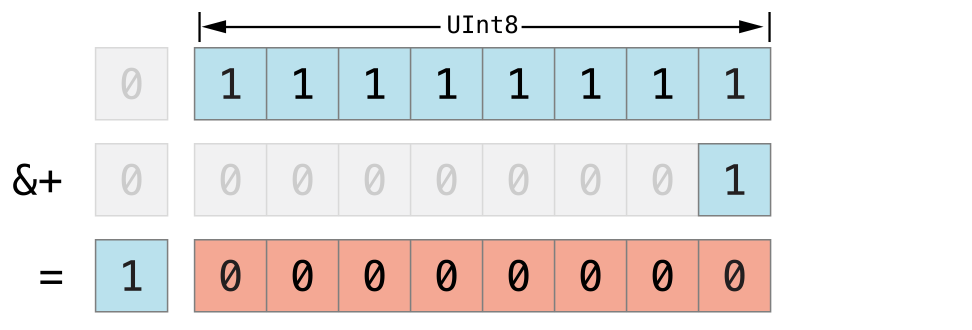``````var unsignedOverflow = UInt8.min
// unsignedOverflow equals 0, which is the minimum value a UInt8 can hold
unsignedOverflow = unsignedOverflow &- 1
// unsignedOverflow is now equal to 255``````

UInt8 型整数能容纳的最小值是  ，以二进制表示即 00000000 。当使用溢出减法运算符（ &- ）对其进行减 1 操作时，数值会产生下溢并被截断为 11111111 ， 也就是十进制数值的 255 。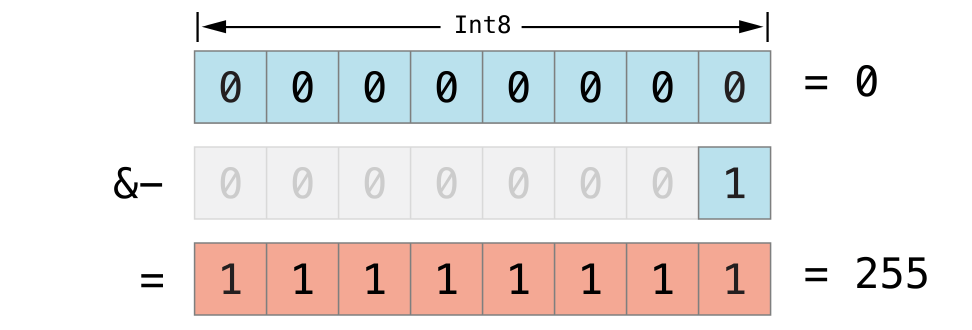``````var signedOverflow = Int8.min
// signedOverflow equals -128, which is the minimum value an Int8 can hold
signedOverflow = signedOverflow &- 1
// signedOverflow is now equal to 127``````

Int8 整数能容纳的最小值是 -128 ，以二进制表示即 10000000 。当使用溢出减法运算符对其进行减 1 操作时，符号位翻转，得到二进制数值 01111111 ，也就是十进制数值的 127 ，这个值也是 Int8  型整数所能容纳的最大值。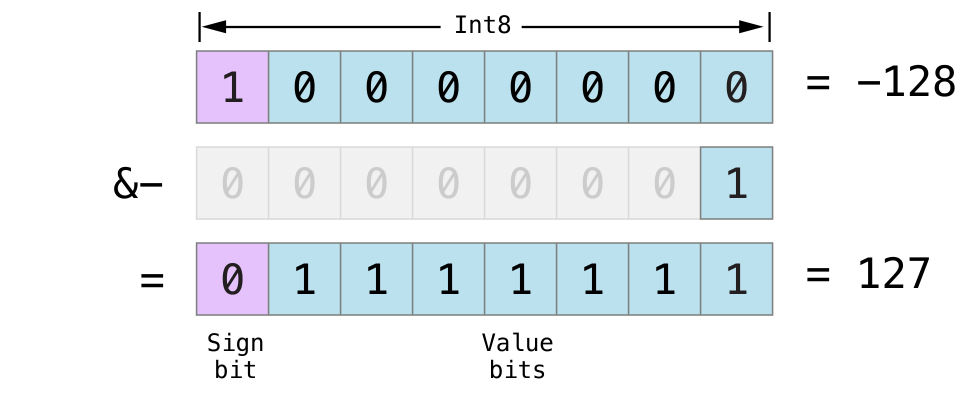## 优先级和结合性

``````2 + 3 % 4 * 5
// this equals 17``````

• 2 + 3 = 5
• 5 % 4 = 1
• 1 5 = 5

``2 + ((3 % 4) * 5)``

(3 % 4) 是 3 ，所以表达式等价于：

``2 + (3 * 5)``

(3 * 5) 是 15 ，所以表达式等价于：

``2 + 15``

## 运算符方法

``````struct Vector2D {
var x = 0.0, y = 0.0
}

extension Vector2D {
static func + (left: Vector2D, right: Vector2D) -> Vector2D {
return Vector2D(x: left.x + right.x, y: left.y + right.y)
}
}``````

``````let vector = Vector2D(x: 3.0, y: 1.0)
let anotherVector = Vector2D(x: 2.0, y: 4.0)
let combinedVector = vector + anotherVector
// combinedVector is a Vector2D instance with values of (5.0, 5.0)``````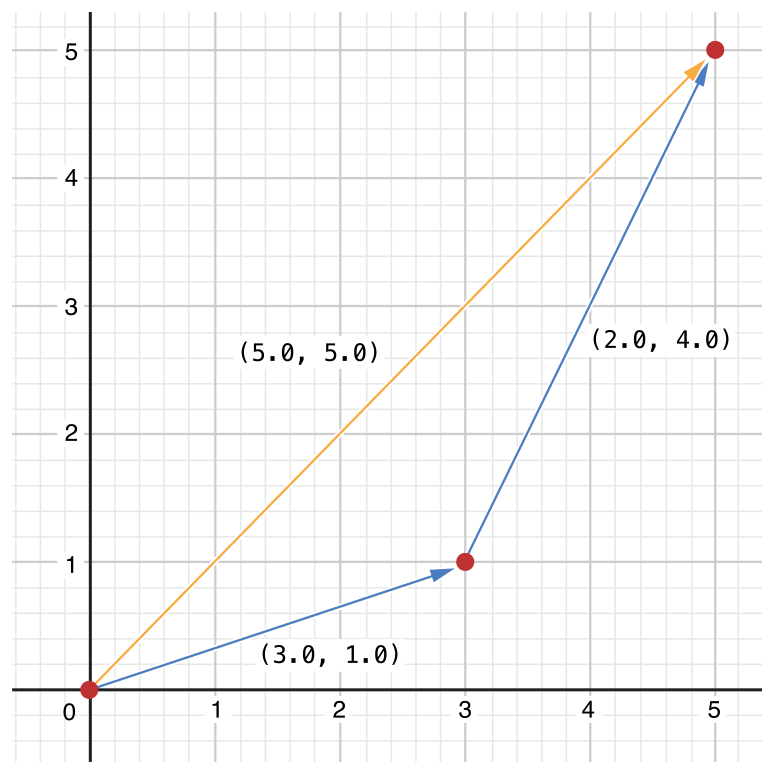### 前缀和后缀运算符

``````extension Vector2D {
static prefix func - (vector: Vector2D) -> Vector2D {
return Vector2D(x: -vector.x, y: -vector.y)
}
}``````

``````let positive = Vector2D(x: 3.0, y: 4.0)
let negative = -positive
// negative is a Vector2D instance with values of (-3.0, -4.0)
let alsoPositive = -negative
// alsoPositive is a Vector2D instance with values of (3.0, 4.0)``````

### 组合赋值运算符

``````extension Vector2D {
static func += (left: inout Vector2D, right: Vector2D) {
left = left + right
}
}``````

``````var original = Vector2D(x: 1.0, y: 2.0)
let vectorToAdd = Vector2D(x: 3.0, y: 4.0)
// original now has values of (4.0, 6.0)``````

### 等价运算符

``````extension Vector2D: Equatable {
static func == (left: Vector2D, right: Vector2D) -> Bool {
return (left.x == right.x) && (left.y == right.y)
}
}``````

``````let twoThree = Vector2D(x: 2.0, y: 3.0)
let anotherTwoThree = Vector2D(x: 2.0, y: 3.0)
if twoThree == anotherTwoThree {
print("These two vectors are equivalent.")
}
// Prints "These two vectors are equivalent."``````

Swift 为以下自定义类型提等价运算符供合成实现：

• 只拥有遵循 Equatable 协议存储属性的结构体；
• 只拥有遵循 Equatable 协议关联类型的枚举；
• 没有关联类型的枚举。

``````struct Vector3D: Equatable {
var x = 0.0, y = 0.0, z = 0.0
}

let twoThreeFour = Vector3D(x: 2.0, y: 3.0, z: 4.0)
let anotherTwoThreeFour = Vector3D(x: 2.0, y: 3.0, z: 4.0)
if twoThreeFour == anotherTwoThreeFour {
print("These two vectors are also equivalent.")
}
// Prints "These two vectors are also equivalent."``````

## 自定义运算符

``prefix operator +++ {}``

``````extension Vector2D {
static prefix func +++ (vector: inout Vector2D) -> Vector2D {
vector += vector
return vector
}
}

var toBeDoubled = Vector2D(x: 1.0, y: 4.0)
let afterDoubling = +++toBeDoubled
// toBeDoubled now has values of (2.0, 8.0)
// afterDoubling also has values of (2.0, 8.0)``````

### 自定义中缀运算符的优先级和结合性

associativity 的默认值是 none ， precedence 默认为 100 。

``````infix operator +- { associativity left precedence 140 }
extension Vector2D {
static func +- (left: Vector2D, right: Vector2D) -> Vector2D {
return Vector2D(x: left.x + right.x, y: left.y - right.y)
}
}
let firstVector = Vector2D(x: 1.0, y: 2.0)
let secondVector = Vector2D(x: 3.0, y: 4.0)
let plusMinusVector = firstVector +- secondVector
// plusMinusVector is a Vector2D instance with values of (4.0, -2.0)``````